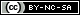# Weibull++ Examples

(Difference between revisions)
 Revision as of 05:30, 22 August 2012 (view source)← Older edit Revision as of 22:35, 23 August 2012 (view source) (→Life Data Analysis)Newer edit → Line 22: Line 22: ::*[[Exponential Distribution Examples|Exponential]] ::*[[Exponential Distribution Examples|Exponential]] ::*[[Normal Distribution Examples|Normal]] ::*[[Normal Distribution Examples|Normal]] - ::*[[Example:_Logistic_Distribution|Logistic]] + ::*[[Logistic_Distribution_Example|Logistic]] - ::*[[Example:_Loglogistic_Distribution|Loglogistic]] + ::*[[Loglogistic_Distribution_Example|Loglogistic]] - ::*[[Example:_Gamma_Distribution|Gamma]] + ::*[[Gamma_Distribution_Example|Gamma]] - ::*[[Example:_Generalized_Gamma_Distribution_Example|Generalized Gamma ]] + ::*[[Generalized_Gamma_Distribution_Example|Generalized Gamma ]] ::*[[Gumbel_Distribution_Example|Gumbel]] ::*[[Gumbel_Distribution_Example|Gumbel]] *[[Weibull++ Non-Parametric LDA Plot Example|Non-Parametric Life Data Analysis (LDA)]] *[[Weibull++ Non-Parametric LDA Plot Example|Non-Parametric Life Data Analysis (LDA)]]

## Quick Start Guide

The Weibull++ & ALTA 8 Quick Start Guide has been designed to help you explore many of the software's key features by working through step-by-step instructions for some practical application examples. The guide is available as a free *.PDF download and you can also watch videos posted on ReliaSoft.tv.

## Life Data Analysis

• Crack Propagation Example (Point Estimation). View it in HTML or Video

## UtilitiesContent on this site is available/licensed under the "Creative Commons Attribution-NonCommercial-ShareAlike 4.0 International License." See Copyright Information for details.